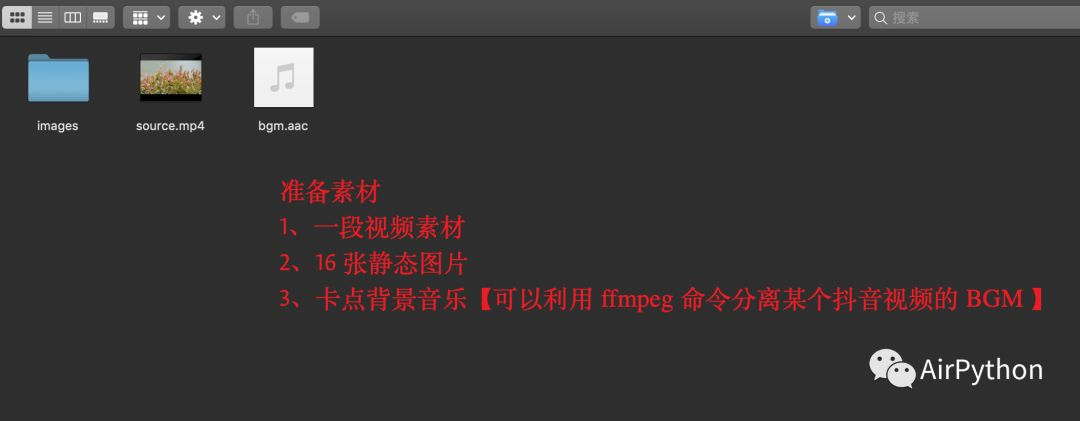# 恋习Python

sldata2017

Today

1

2

``# opencv 用于剪辑视频pip3 install opencv-python``

``# 分离BGM、合并视频和BGMpip3 install ffmpeg``

3``# 视频源videoCapture = cv2.VideoCapture(soure_filename)# 获取视频的帧率fps = videoCapture.get(cv2.CAP_PROP_FPS)# 获取视频的分辨率img_size = (int(videoCapture.get(cv2.CAP_PROP_FRAME_WIDTH)),                int(videoCapture.get(cv2.CAP_PROP_FRAME_HEIGHT)))``

``# 构建一个视频写入对象video_writer = cv2.VideoWriter(output_filename, cv2.VideoWriter_fourcc('X', 'V', 'I', 'D'), fps, img_size)``

``# 开始帧和结束帧start_frame = fps * start_timeend_frame = start_frame + peroid * fps# 循环读取视频帧，只写入开始帧和结束帧之间的帧数据while True:      success, frame = videoCapture.read()        if success:            i += 1            if start_frame <= i <= end_frame:                # 将截取到的画面写入“新视频”                video_writer.write(frame)      else:            break# 释放资源videoCapture.release()``

ps：为了保证每一张静态图片都显示 0.5s，并且静态图片生成的视频与第一段视频使用同一帧率，因此我们应该针对每一张图片写入多次，即多帧数据。每张图片写入的总次数为上段视频的帧率的 1/2。

``# 视频格式：MP4fourcc = cv2.VideoWriter_fourcc('m', 'p', '4', 'v')# 构建写入对象video = cv2.VideoWriter(output_video_path, fourcc, fps, img_size)# 每一张图片要写入的帧数目total_count = math.ceil(fps / 2)``

``def resize_image(target_image_path, target_size):    """    调整图片大小，缺失的部分用黑色填充    :param target_image_path: 图片路径    :param target_size: 分辨率大小    :return:    """    image = Image.open(target_image_path)    iw, ih = image.size  # 原始图像的尺寸    w, h = target_size  # 目标图像的尺寸    scale = min(w / iw, h / ih)  # 转换的最小比例    # 保证长或宽，至少一个符合目标图像的尺寸    nw = int(iw * scale)    nh = int(ih * scale)    image = image.resize((nw, nh), Image.BICUBIC)  # 缩小图像    # image.show()    new_image = Image.new('RGB', target_size, (0, 0, 0, 0))  # 生成黑色图像    # 将图像填充为中间图像，两侧为灰色的样式        new_image.paste(image, ((w - nw) // 2, (h - nh) // 2))      # 覆盖原图片    new_image.save(target_image_path)``

``# 使用opencv读取图像frame = cv2.imread(image_path)# 直接缩放到指定大小frame_suitable = cv2.resize(frame, (img_size, img_size), interpolation=cv2.INTER_CUBIC)# 把图片写进视频# 重复写入多少次count = 0while count < total_count:       video.write(frame_suitable)       count += 1``

``ret, frame = cap.read()while ret:     # 文字在图中的坐标(注意:这里的坐标原点是图片左上角)     x, y = img_size - 200, img_size - 50     # 写入水印文字，文字颜色为白色     cv2.putText(img=frame, text=mask_word,                    org=(x, y), fontFace=cv2.FONT_HERSHEY_COMPLEX_SMALL,                    fontScale=1, color=(255, 255, 255))     video_writer.write(frame)     ret, frame = cap.read()# 删除源文件，并重命名临时文件os.remove(video_path)os.rename(video_temp_path, video_path)print('水印添加完成~')video_writer.release()cap.release()``

``#获取视频的长度cap = cv2.VideoCapture(video_path)#帧率fps = cap.get(cv2.CAP_PROP_FPS)#总帧数frame_count = cap.get(cv2.CAP_PROP_FRAME_COUNT)#视频总时长-秒，这里做取整操作 【浮点类型】time_count = math.floor(frame_count / fps)print('帧率:%f,总帧数:%d' % (fps, frame_count))print(time_count)# 3.截取音频# 为了简单，这里一般不会超过一分钟bgm_temp_path = get_temp_path(bgm_path, 'temp_new')os.system('ffmpeg -i %s -ss 00:00:00 -t 00:00:%d -acodec copy %s' % (bgm_path, time_count, bgm_temp_path))``

``#视频、音频合二为一# 临时文件video_temp_path = get_temp_path(video_path, 'temp')os.system('ffmpeg -i %s  -i %s  -vcodec copy -acodec copy %s' % (video_path, bgm_path, video_temp_path))# 删除源文件，重命令临时文件os.remove(video_path)os.rename(video_temp_path, video_path)``

4

::...#### 自怼圈/年度番新